Vol. 13
Latest Volume
All Volumes
All Journals
2010-08-20
PIER M
Vol. 13, 261-273, 2010
A Planar Focusing Antenna Design with the Quasi-Conformal Mapping
Zhong-Lei Mei , Jing Bai , Tiao Ming Niu and Tie-Jun Cui
We propose a planar focusing antenna design, which has the same performance as its parabolic counterparts and can be realized using PEC-backed gradient index dielectrics. In this design, quasi-conformal transformation optics is first utilized to transform a parabolic surface into a planar one, then the anisotropy factor of the resultant material is minimized, and the material is approximately treated as isotropic. Examples with realizable material parameters are given, and the simulation results validate the design. The proposed method could be used to design planar focusing antennas with high directivity and similar devices. The idea can also be applied to new device designs in optics engineering.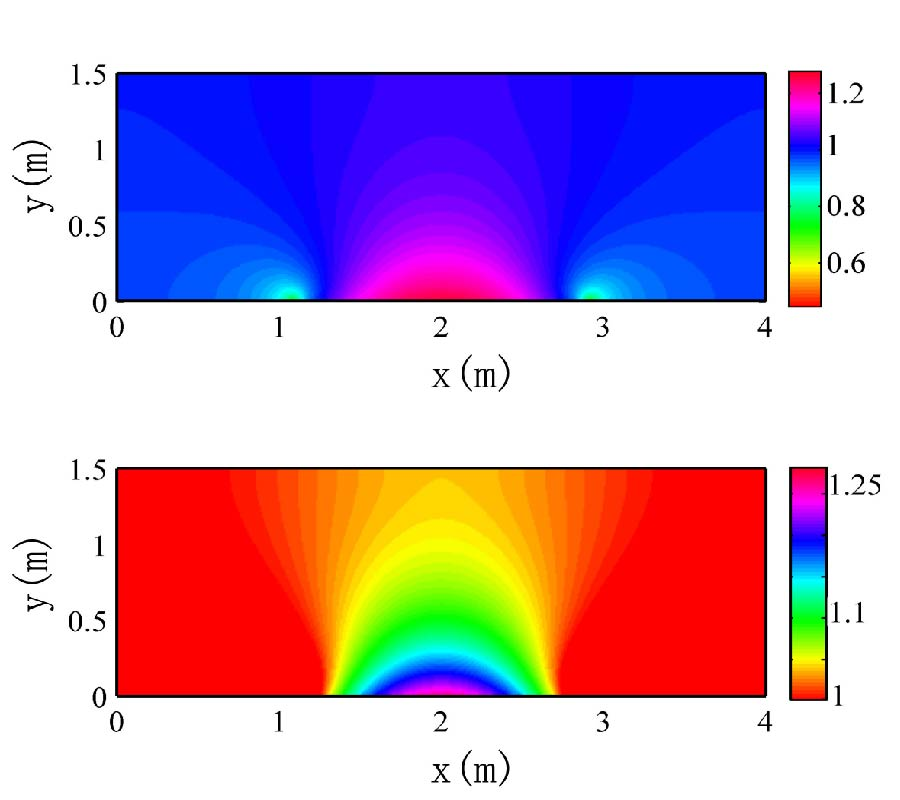2010-08-19
PIER M
Vol. 13, 245-260, 2010
A New Method for Calculating Transient Electromagnetic Responses of ac /DC Power System with External Electromagnetic Pulse Interference
Xiao-Yun Huo and Yin-Zhao Lei
In this paper, a new method for calculating transient electromagnetic responses of AC/DC power system with external electromagnetic pulse interference is proposed. An input-output model of three-phase bridge rectifier is presented for the transient calculation. In order to study the effect of the external electromagnetic pulse on the system, the field-to-line coupling model is introduced, and finite-difference time-domain method is adopted. Thus, the modeling method utilizes the analysis methods of the electric circuits and electromagnetic fields synthetically to deal with the coupled field-circuit problems. The model and algorithm are validated by comparing the calculation results with the experiment ones. Finally, the effects of some circuit parameters on transient responses are discussed. The method proposed in this paper lays the foundation for further researches on the transient electromagnetic performance of independent electrical power systems containing power electronics.2010-08-17
PIER M
Vol. 13, 229-243, 2010
FDTD Modeling and Simulation of Microwave Heating of in-Shell Eggs
Satyanarayan R. S. Dev , Yvan Gariepy , Valerie Orsat and Vijaya G. S. Raghavan
Considering microwaves as a viable alternative for the pasteurization of In-shell eggs, preliminary trials performed had confirmed that microwave at 2450 MHz can be successfully used to raise the temperature of in-shell eggs to the required pasteurization temperatures in a few minutes. Based on these trials a finite difference time domain (FDTD) model was developed using C language and MATLAB to simulate the E field and power distribution in lossy dielectric media like that of the egg components (egg white and yolk) taking into consideration the complex shape, dielectric properties and heterogeneous composition of the in-shell egg. This can be used to assist in the design and development of an industrial microwave in-shell eggs pasteurization unit.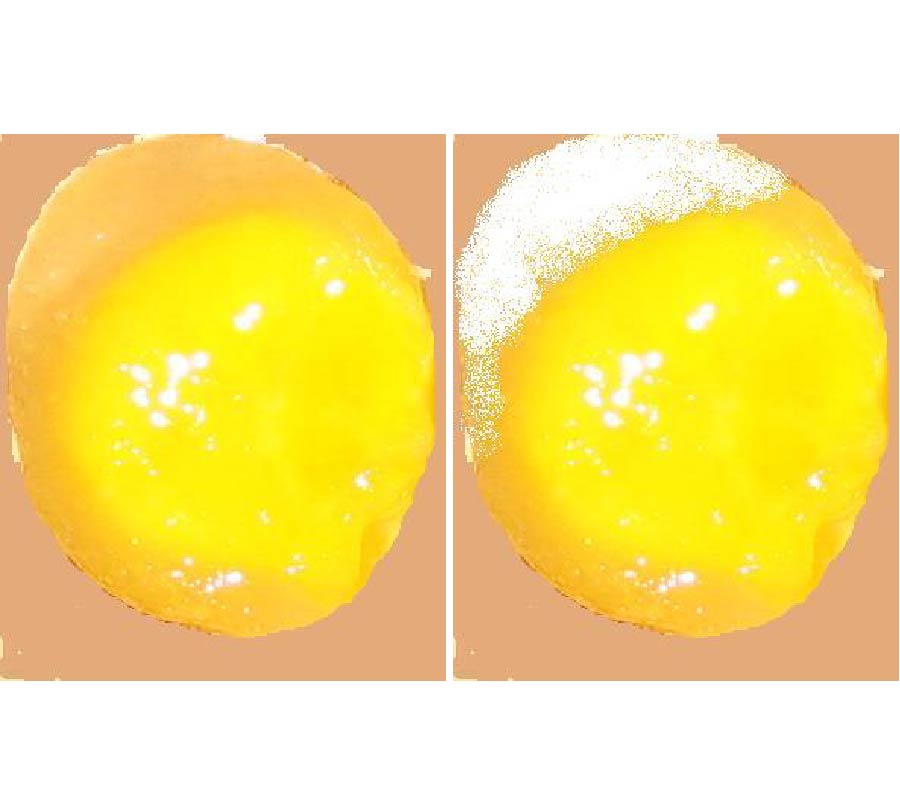2010-08-17
PIER M
Vol. 13, 217-227, 2010
Analysis of Characteristics of Two-Dimensional Runge-Kutta Multiresolution Time-Domain Scheme
Qunsheng Cao and Xinlei Chen
In this paper the stability condition of the Runge-Kutta m-order multiresolution time-domain (RKm-MRTD) scheme has been studied. By analyzing the amplification factors, we derive the numerical dispersion relation of the RK-MRTD scheme. The numerical dispersive and dissipative errors are investigated. Finally, the theoretical predictions of the numerical errors are calculated through the numerical simulations.2010-08-16
PIER M
Vol. 13, 203-215, 2010
Electromagnetic Wave Scattering by Many Small Bodies and Creating Materials with a Desired Refraction Coefficient
Alexander G. Ramm
Electromagnetic wave scattering by many small particles is studied. An integral equation is derived for the self-consistent field E in a medium, obtained by embedding many small particles into a given region D. The derivation of this integral equation uses a lemma about convergence of certain sums. These sums are similar to Riemannian sums for the integral equation for E. Convergence of these sums is essentially equivalent to convergence of a collocation method for solving this integral equation. By choosing the distribution law for embedding the small particles and their physical properties one can create a medium with a desired refraction coefficient. This coefficient can be a tensor. It may have a desired absorption properties.
2010-08-10
PIER M
Vol. 13, 189-202, 2010
Analytic Expression for the Effective Plasma Frequency in One-Dimensional Metallic-Dielectric Photonic Crystal
Jesus Manzanares-Martinez
In this work, an analytic expression to define the effective plasma frequency of an one-dimensional periodic system containing alternating dielectric and metallic slabs is proposed. Such metallic elements are considered to have a Drude dielectric function. The effective plasma frequency is obtained as a simple average of the constitutive materials, and its cutoff frequency for the propagating modes is compared with band structure calculations. We also explore the role of absorption in the transparency frequency cutoff.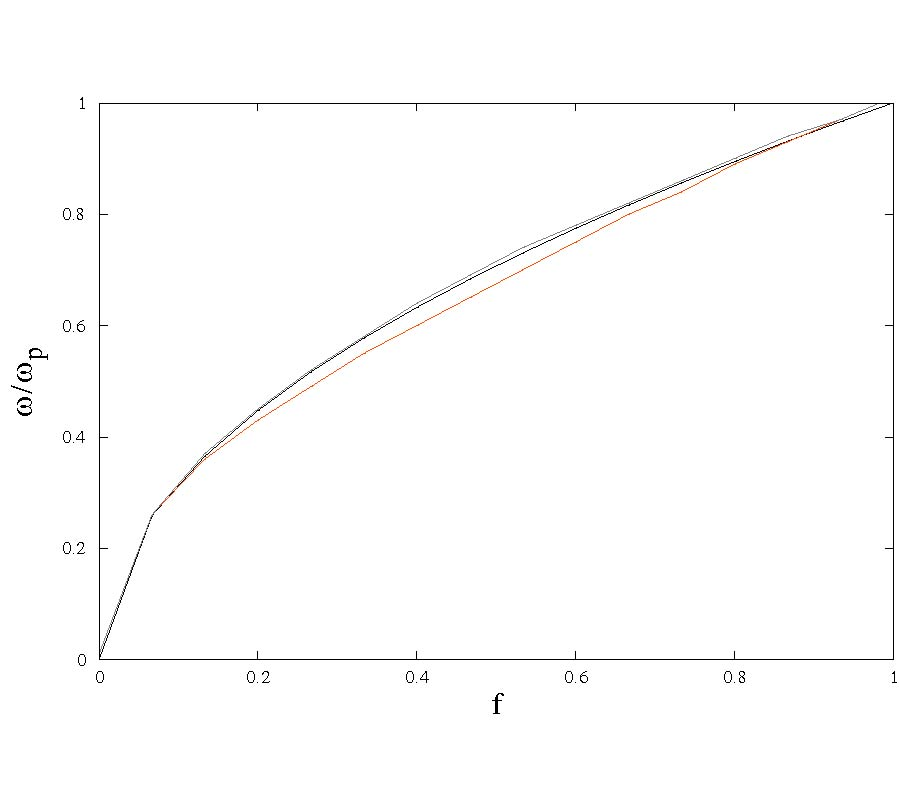2010-08-02
PIER M
Vol. 13, 173-188, 2010
Artificial Neural Networks Approach in Microwave Filter Tuning
Jerzy Julian Michalski
This paper presents a novel method of cavity filter tuning with the usage of an artificial neural network (ANN). The proposed method does not require information on the filter topology, and the filter is treated as a black box. In order to illustrate the concept, a feed-forward, multi-layer, non-linear artificial neural network with back propagation is applied. The method for preparing, learning and testing vectors consisting of sampled detuned scattering characteristics and corresponding tuning screw deviations is proposed. To collect the training vectors, the machine, an intelligent automatic filter tuning tool integrated with a vector network analyzer, has been built. The ANN was trained on the basis of samples obtained from a properly tuned filter. It has been proved that the usage of multidimensional approximation ability of an ANN makes it possible to map the characteristic of a detuned filter reflection in individual screw errors. Finally, after the ANN learning process, the tuning experiment on 6 and 11-cavity filters has been preformed, proving a very high efficiency of the presented method.2010-07-29
PIER M
Vol. 13, 157-171, 2010
Leaky-Wave Regimes on MEMS-Loaded Transmission Lines for mm -Wave Applications
Tomas Zvolensky , Dmitry Chicherin , Antti V. Räisänen and Constantin R. Simovski
This paper presents study of controllable leaky wave modes in various planar transmission lines operating at millimetre wavelengths. Leaky wave regime is achieved by exploitation of periodic inclusions. The main goal is to obtain the scanning of the radiation angle from forward to backward direction and rather broad range of scanning angles at a given operation frequency corresponding to the mm-wave range. For this purpose we suggest to use MEMS capacitors combined with shunt strap inductors, probably grounded. This design solution allows one to significantly reduce the losses in the loaded line compared to known scanning leaky-wave antennas based on varactors or on magnetized ferrites. The design of the unit cell is done using global optimization method, and the dispersion is investigated analytically. After analytical modeling and optimization, full wave analysis is done using Ansoft HFSS v.11 environment. After the leaky wave regimes are verified, an example of a leaky-wave antenna is introduced in order to confirm possibility of beam scanning.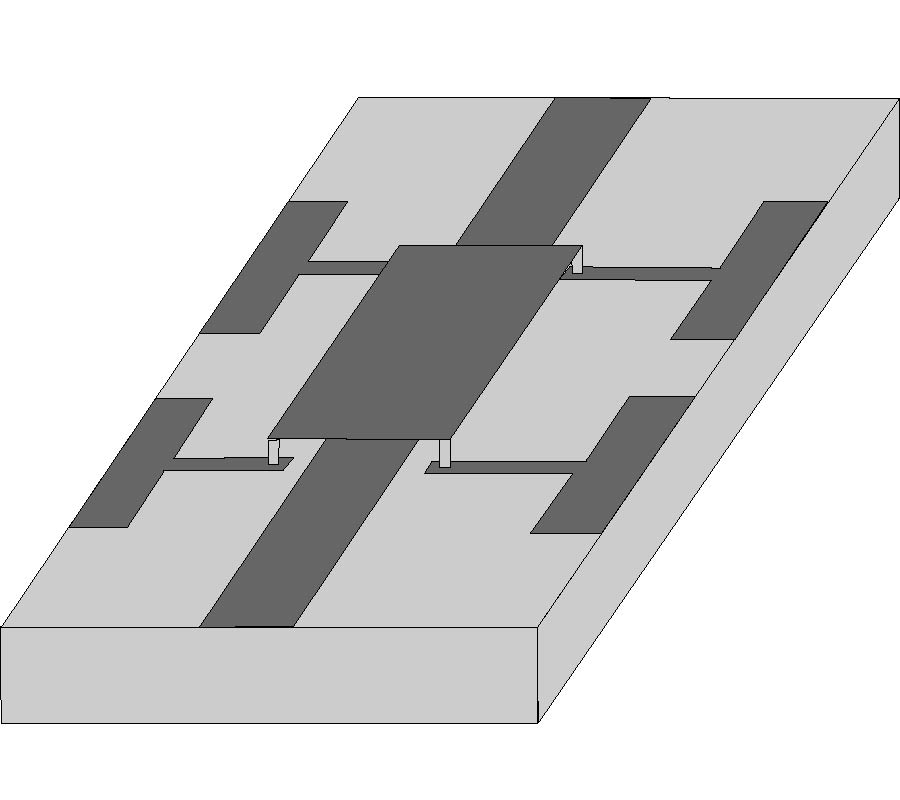2010-07-25
PIER M
Vol. 13, 145-156, 2010
Covariant Constitutive Relations, Landau Damping and Non-Stationary Inhomogeneous Plasmas
Jonathan Gratus and Robin W. Tucker
Models of covariant linear electromagnetic constitutive relations are formulated that have wide applicability to the computation of susceptibility tensors for dispersive and inhomogeneous media. A perturbative framework is used to derive a linear constitutive relation for a globally neutral plasma enabling one to describe in this context a generalized Landau damping mechanism for non-stationary inhomogeneous plasma states.2010-07-14
PIER M
Vol. 13, 133-143, 2010
Higher Order Hierarchical Legendre Basis Functions Application to the Analysis of Scattering by Uniaxial Anisotropic Objects
Chaojie Lv , Yan Shi and Chang-Hong Liang
An efficient technique for the analysis of scattering by uniaxial anisotropic objects is presented. The technique is based on the method of higher order MoM of the surface integral equations. This higher order MoM solution uses the higher order hierarchical basis functions which are based on the modified Legendre polynomials. Numerical results are given to demonstrate that the higher order hierarchical basis functions are more accurate and efficient in the calculations of uniaxial anisotropic objects scattering problem than the low-order basis function.2010-07-13
PIER M
Vol. 13, 121-131, 2010
Full-Wave Analysis of Dielectric Rectangular Waveguides
Jigyasa Sharma and Asok De
In this paper the characteristic equations of the Eymn and Exmn modes of the dielectric rectangular waveguide have been derived using the mode matching technique. No assumptions have been taken in the derivations which have been straight forwardly done. Two ratios have been introduced in the characteristic equations and the new set of characteristic equations thus obtained are then plotted and graphical solutions are obtained for the propagation parameters assuming certain numerical values for the introduced ratios. The results have then been compared to those obtained by Marcatilli and Goell for rectangular dielectric waveguides. The comparisons depict a good agreement in the three methods at frequencies well above cut-off.2010-07-12
PIER M
Vol. 13, 109-119, 2010
Parameter Extraction for Microwave Coupled Resonator Filters Using Rational Model and Optimization
Jie Peng , Bian Wu , Chang-Hong Liang and Xue-Feng Li
A method is presented for the parameter extraction of microwave coupled resonator filters. The method is based on the estimation of a rational model of the filters. From these rational functions, a circuit network having the previously know topology is optimized. Two simple and efficient error functions are used to reduce the computational effort of the optimization while improving the speed and robustness of diagnosis process for lossless and lossy filters, respectively. Two numerical examples are presented to demonstrate the efficiency of the proposed technique. One deals with numerical simulation data from a full-wave electromagnetic simulation and the other one uses the measured data.2010-07-09
PIER M
Vol. 13, 95-108, 2010
SAR and Temperature Elevation in a Multi-Layered Human Head Model Due to an Obliquely Incident Plane Wave
Ayman I. Sabbah , Nihad I. Dib and Moh'd A. Al-Nimr
In this paper, the Specific Absorption Rate (SAR) distribution in a human head irradiated by an obliquely incident electromagnetic plane wave is studied. A simple planar multi-layered structure is used to model the human head. Moreover, the steady state thermal elevation distribution is calculated by solving the bioheat equation using the finite difference time domain (FDTD) method. Both types of wave polarization (perpendicular and parallel) will be discussed. The obtained results confirm the importance of performing a thermal analysis along with the dosimetric one. It is found that the induced temperature elevation in the brain region, in all the examined conditions, never exceeds 0.4℃. This value is well below the threshold for the induction of adverse thermal effects to the neurons which is 3.5℃.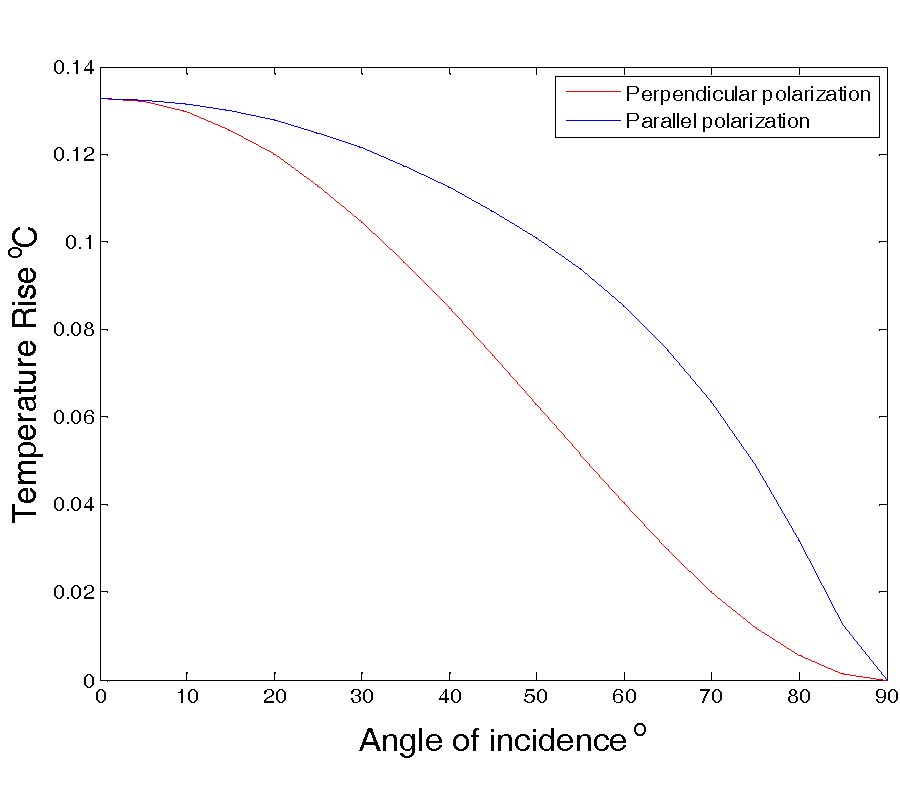2010-07-07
PIER M
Vol. 13, 83-94, 2010
Analytical Pole Residue Calculation in Spectral Method of Moments Formulations for Periodic Structures
Y. Kaganovsky and Reuven Shavit
An analytical method for evaluating pole-residues in spectral method of moments (MoM) formulations is presented. Spectral integral formulations for periodic structures involve the inverse of the MoM matrix, which exhibits a periodic set of pole singularities, corresponding to the zeros of the matrix's determinant. So far, these singularities have not been extracted and the corresponding pole-residues were calculated directly from the differential or integral definitions of the residue. In this work, we consider an analytical expression for the solution to the MoM matrix equation, which enables the extraction of pole singularities and the analytical evaluation of pole-residues. We also present a comparison to previous methods.2010-07-07
PIER M
Vol. 13, 69-82, 2010
Loss of Linear Momentum in an Electrodynamics System: from an Analytical Approach to Simulations
Dimitri S. H. Charrier
The classical electrodynamics allows the use of retarded electromagnetic fields. The purpose of this investigation is to predict the useful force in a coil-ring system by electromagnetomechanical conversion. Analytical equations in retarded regime are given and are used to simulate a realistic thruster based on the coil-ring system. It is shown that a net force in the inertial coil-ring system is created. By means of high frequency electronics, analytical and practical tools toward new experiments for electromagnetic thrusters are given.2010-06-26
PIER M
Vol. 13, 53-68, 2010
Broadband Measurement of Complex Permittivity of Composite at Microwave Frequencies Using Scalar Scattering Parameters
Subasit Borah and Nidhi Saxena Bhattacharyya
A shielded, conductor-backed coplanar waveguide technique is used to determine the complex permittivity and loss tangent of nano magnetic composite materials over X-band. The test composite material is synthesized by reinforcing cobalt ferrite particles with average crystallite diameter 7.36nm in low density polyethylene matrix with 2% and 4% volume fractions. The complex permittivity for low density polyethylene matrix and the composite samples, evaluated from the present technique at 9.887 GHz, are verified with cavity perturbation technique resonating at the same frequency. A new mathematical approach, using element-to-element correspondence of the ABCD matrix, is applied to calculate the complex propagation constant. The formulation facilitates evaluation of complex propagation constant over the test frequency range using scalar scattering parameters without altering the coplanar waveguide geometry. The mathematical formulation is verified by performing permittivity measurements for air over the X-band.2010-06-22
PIER M
Vol. 13, 41-51, 2010
Analysis of Terahertz-Induced Optical Phase Modulation in a Nonlinear Dielectric Slab
Frequency shift of the spectrum of an incident optical pulse by an intense THz pulse inducing cross-phase modulation (XPM) in a nonlinear dielectric slab is analyzed. The effect is predicted with a high degree of accuracy using the well-known transmission line matrix (TLM) technique. In this research, to model the THzinduced temporal and spatial variation of the dielectric permittivity of the nonlinear dielectric slab, the transmission lines of the TLM method are loaded with open shunt stubs. The parameters of the stubs are modified in accordance with the refractive index variation of the dielectric slab, here ZnTe, induced by the strong THz pulse. The obtained numerical results are verified with a recently reported experimental work.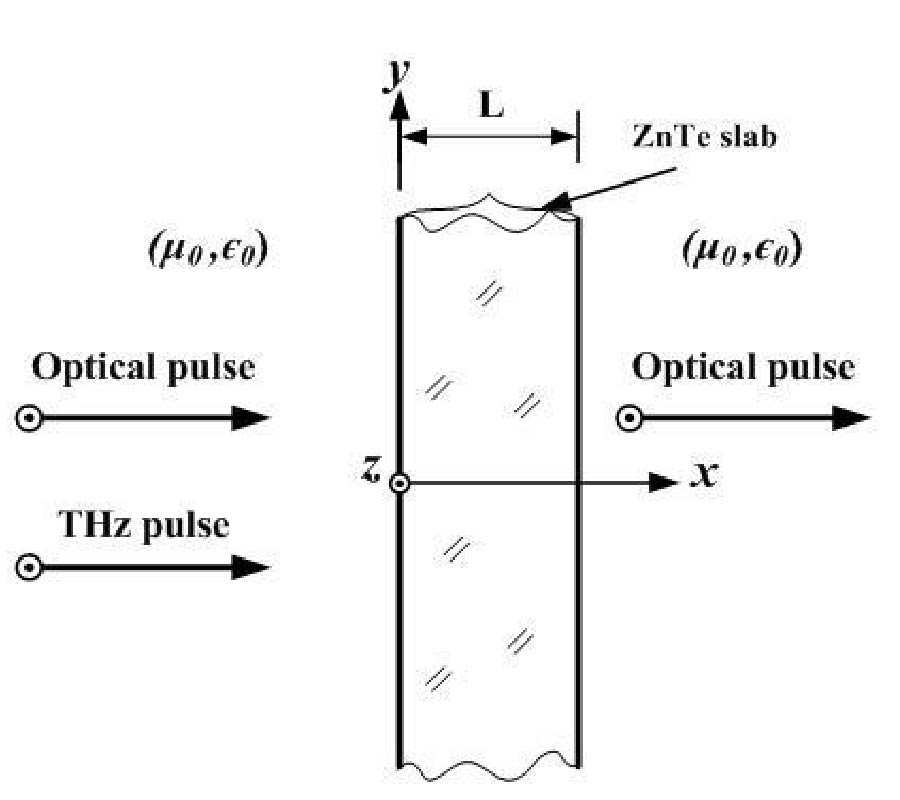2010-06-16
PIER M
Vol. 13, 29-40, 2010
Shift-Operator Finite Difference Time Domain Analysis of Chiral Medium
Ahmed Attiya
Shift-Operator Finite difference Time Domain (SO-FDTD) method is introduced as a new efficient technique for simulating electromagnetic wave interaction with chiral medium. The dispersive properties of this medium are presented as polynomials of . These polynomials are converted to time domain by replacing by the time derivative operator. Then this time derivative operator is converted to the corresponding time shift operator which is used directly to obtain the corresponding update equations of electric and magnetic field components. The resulting update equations do not require time convolution or additional vector components. The present analysis does not require also any transformation. Significant improvement is obtained in memory requirements by using this method while the computational time is nearly the same compared with other similar techniques like Z-transformation FDTD.2010-06-16
PIER M
Vol. 13, 17-28, 2010